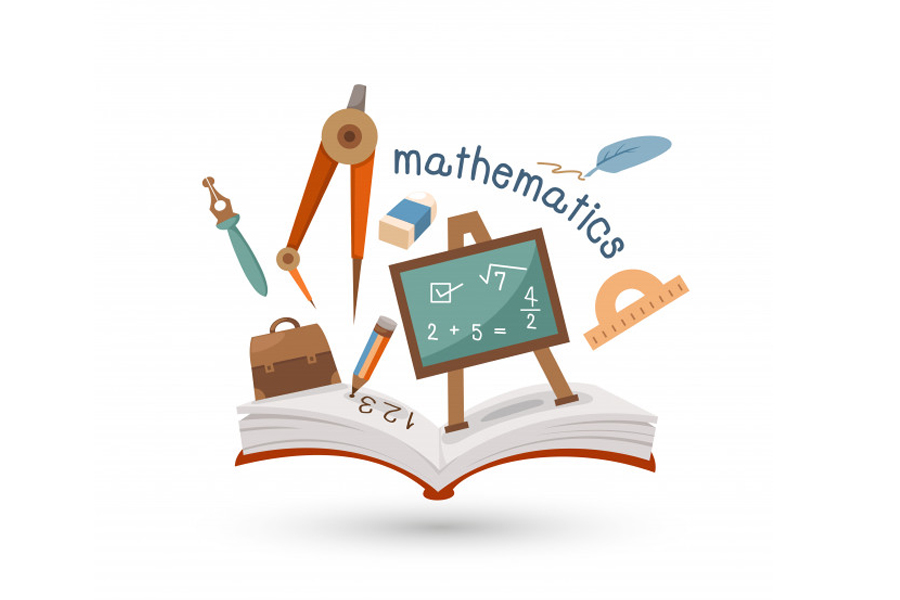## Elementary Math Grades 3 - 5[PL][8-10]## Private Lessons

• 2 sessions every week
• Each session is of 1 hour

#### Overview

Aligned with the common core curriculum of 3rd to 5th grades, these sessions focus on building on the basics children learned in the lower grades. These sessions teach and help kids practice advanced concepts of multiplication, division, measurement and geometry.

#### Curriculum

###### G3-1 Number and Operations in Base 10 - 3 2 hrs

• 1 Place Value and Rounding
• 2 Rounding to the nearest 10 or 100
• 3 3 digit and 4 digit addition and subtraction
• 4 Multiply one-digit whole numbers by multiples of 10

###### G3-2 Operations & Algebraic Thinking - 3 4 hrs

• 5 Multiplication: properties of multiplication, grouping, multiply within 100
• 6 Division: relationship between multiplication and division, divide within 100
• 7 Solve two-step word problems using the four operations
• 8 Identify arithmetic patterns

###### G3-3 Numbers & Operations - Fractions - 3 4 hrs

• 9 Understanding Fractions
• 10 Fraction as a number on the number line
• 11 Comparing Fractions
• 12 Decimals

###### G3-4 Measurement & Data - 3 4 hrs

• 13 Time: Tell time to the nearest minute, addition and subtraction of time intervals
• 14 Geometric Measurement: Perimeter, Area, Volume and Capacity
• 15 Represent and Interpret Data: Scaled picture graph, scaled bar graph, making line plots

###### G3-5 Geometry - 3 4 hrs

• 16 Shapes in different categories: Triangles, Quadrilaterals
• 17 Quadrilaterals with different orientations
• 18 Partitioning shapes

###### G4-1 Operations and Algebraic Thinking - 4 4 hrs

• 19 Use the four operations with whole numbers
• 20 Factors and Multiples
• 21 Number Patterns

###### G4-2 Number & Operations in Base Ten - 4 4 hrs

• 22 Place value understanding of multi-digit whole numbers
• 23 Round multi-digit whole numbers to any place
• 24 Multi digit addition, subtraction, multiplication and division

###### G4-3 Numbers & Operations - Fractions - 4 4 hrs

• 25 Fraction equivalents and comparison
• 26 Mixed numbers as fractions
• 27 Addition and subtraction of fractions and mixed numbers
• 28 Multiplication of fraction by a whole number
• 29 Decimal notations for fractions, compare decimal fractions

###### G4-4 Measurement & Data - 4 4 hrs

• 30 Relative size of measurement
• 31 Use the four operations to solve word problems involving distances, intervals of time, liquid volumes, masses of objects, and money
• 32 Area and Perimeter: Real world problems
• 33 Line plot of measurements in fractions
• 34 Understanding Angles
• 35 Measure, add, subtract Angles

###### G4-5 Geometry - 4 4 hrs

• 36 Lines, line segments, rays, angles
• 37 Classify two dimensional figures
• 38 Lines of Symmetry

###### G5-1 Number & Operations in Base Ten - 5 4 hrs

• 39 Decimal place value
• 40 Pattern in the number of zeros and placement of decimal point
• 41 Represent decimals in expanded form
• 42 Round decimals to any place
• 43 Multiply multi-digit whole numbers using the standard algorithm
• 44 Find whole-number quotients of whole numbers with up to four-digit dividends and two-digit divisors
• 45 Add, subtract, multiply, and divide decimals to hundredths

###### G5-2 Operations and Algebraic Thinking - 5 4 hrs

• 46 Write and Interpret Expressions
• 47 Analyze Patterns & Relationships

###### G5-3 Numbers & Operations - Fractions - 5 4 hrs

• 48 Use equivalent fractions as a strategy to add and subtract fractions
• 49 Multiplication and Division with Fractions
• 50 Interpret multiplication and division as scaling / resizing
• 51 Real world problems with Fractions

###### G5-4 Measurement & Data - 5 4 hrs

• 52 Convert measurement units
• 53 Represent and interpret data: line plots
• 54 Geometric measurement: understand concepts of volume

###### G5-5 Geometry - 5 4 hrs

• 55 Graph points on the coordinate plane
• 56 2 Classify two-dimensional figures
• 57 2 Hierarchy of two-dimensional figures

### Enroll for Math Private LessonsEnroll today to book your slot!

*Weekly 2 classes. Only limited seats available, Enroll Today.